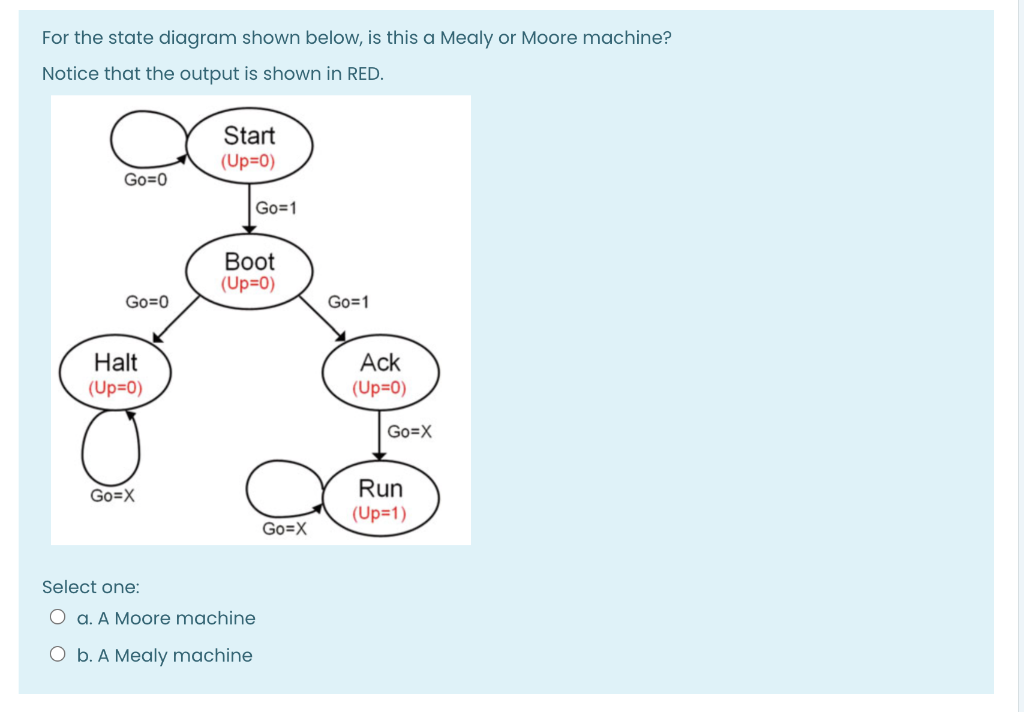# Question URGENT For the state diagram shown below, is this a Mealy or Moore machine? Notice that the output is shown in RED. Start (Up=0) Go=0 Go=1 Boot (Up=0) Go=0 Go=1 Halt (Up=0) Ack (Up=0) Go=X Go=X Run (Up=1) Go=X Select one: O a. A Moore machine O b. A Mealy machineZ0HT1U The Asker · Computer ScienceURGENT

Transcribed Image Text: For the state diagram shown below, is this a Mealy or Moore machine? Notice that the output is shown in RED. Start (Up=0) Go=0 Go=1 Boot (Up=0) Go=0 Go=1 Halt (Up=0) Ack (Up=0) Go=X Go=X Run (Up=1) Go=X Select one: O a. A Moore machine O b. A Mealy machine
More
Transcribed Image Text: For the state diagram shown below, is this a Mealy or Moore machine? Notice that the output is shown in RED. Start (Up=0) Go=0 Go=1 Boot (Up=0) Go=0 Go=1 Halt (Up=0) Ack (Up=0) Go=X Go=X Run (Up=1) Go=X Select one: O a. A Moore machine O b. A Mealy machine